Choose Language Hide Translation Bar
Highlighted

## Add column from one table to another table

I have two data tables ("Table_A" and "Table_B"), both of which have a column "ID". They also both contain a column "Data_1", however with different values. In addition, Table_B has a column "Data_2".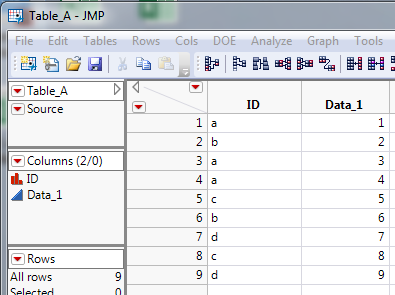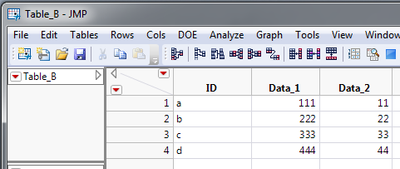I would like to add Data_2 from Table_B to Table_A by matching the ID columns, however, Data_1 in Table_A should NOT be updated with the values of Data_1 in Table_B. This is the desired result: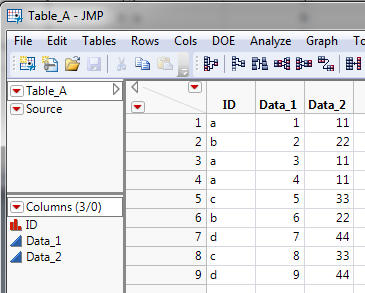The only way I have found to achieve this is by using the Join command, by giving the particular output columns I require: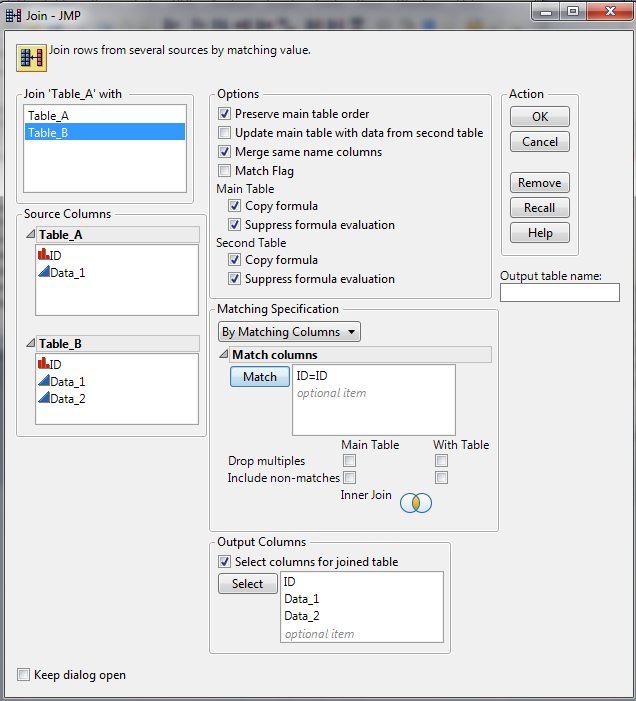However, this creates a new table. I would prefer Data_2 from Table_B to simply be added to Table_A, without any further modifications to Table_A (which is why I cannot use Update). Is there a way to achieve this?

1 ACCEPTED SOLUTION

Accepted Solutions
Highlighted

## Re: Add column from one table to another table

You can use UPDATE, but you have to remove the DATA_1 column from table B first.  Here's some sample code that does this via SUBSET.

JMP Development - this looks like a bug in the UPDATE command; i.e. it's updating Table A with the Data_1 column from Table B even though I didn't ask it to.

dta = New Table( "TableA", Add Rows( 9 ),

New Column( "ID", Character, Nominal,

Set Values( {"a", "b", "a", "a", "c", "b", "d", "c", "d"} ) ),

New Column( "Data_1", Numeric, Continuous, Format( "Best", 12 ),

Set Values( [1, 2, 3, 4, 5, 6, 7, 8, 9] ) )

);

dtb = New Table( "TableB", Add Rows( 4 ),

New Column( "ID", Character, Nominal, Set Values( {"a", "b", "c", "d"} ) ),

New Column( "Data_1", Numeric, Continuous, Format( "Best", 12 ),

Set Values( [111, 222, 333, 444] ) ),

New Column( "Data_2", Numeric, Continuous, Format( "Best", 12 ),

Set Values( [11, 22, 33, 44] ) )

);

dtb_subset = Data Table( "TableB" ) << Subset( All rows, columns( :ID, :Data_2 ) );

dta << Update(

With( dtb_subset ),

Match Columns( :ID = :ID ),

Add Columns from Update table( :Data_2 )

);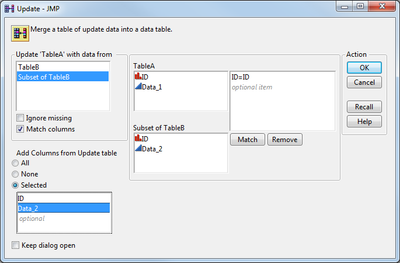10 REPLIES 10
Highlighted

## Re: Add column from one table to another table

If you unselect the Merge Same Name Columns, JMP will join the 2 tables placing all columns independently into the new data table, while still matching on your matching column(s)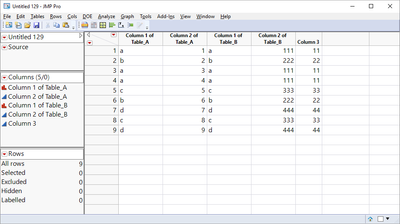Jim
Highlighted

## Re: Add column from one table to another table

Hi Jim,

However, like this I'd end up with several columns that I don't require and which I'd have to delete after the join (my actual table is more complex, above example is obviously simplified, so I'd have to remove quite a few columns).

I'm just finding it hard to believe there's no elegant way to do something as simple as "copy one column only from a data table to another table, matching a certain other column".

Highlighted

## Re: Add column from one table to another table

Hi gandi2223

Try this option to see if it works for you.

``````dtA = New Table( "TableA",
New Column( "ID", Character, Nominal, Set Values( {"a", "b", "c", "d", "e", "f", "g", "h", "i", "j", "k", "l"} ) ),
New Column( "Data1", Numeric, Continuous, Format( "Best", 12 ), Set Values( [1, 2, 3, 4, 5, 6, 7, 8, 9, 10, 11, 12] ) ),

);

dtB = New Table( "TableB",
New Column( "ID", Character, Nominal, Set Values( {"a", "b", "c", "d", "e", "f", "g", "h", "i", "j", "k", "l"} ) ),
New Column( "Data1", Numeric, Continuous, Format( "Best", 12 ), Set Values( [11, 22, 33, 44, 55, 66, 77, 88, 99, 111, 112, 123] ) ),
New Column( "Data2", Numeric, Continuous, Format( "Best", 12 ), Set Values( [10, 20, 30, 40, 50, 60, 70, 80, 90, 100, 110, 120] ) ),

);

// duplicate table B befor removing column "Data1"
dtC = dtB << Subset( All rows , /*private*/ );  // private is just for speed and memory

// remove column "Data1"
dtC << Delete Column( Data1 );

// alternativly you can use this generic column deleteing method
columnstokeep = {"ID", "Data2" /* , "Data3", "Data4"*/ };
columnstotrash = {};
For( icol = 1, icol <= N Col( dtC ), icol++,
colname = Column( dtC, icol ) << get name();
If( Contains( columnstokeep, colname ) < 1,
Insert Into( columnstotrash, colname )
);
);
dtC << delete columns( columnstotrash );

// now update the tables
dtA << Update( With( dtC ), Match Columns( :ID = :ID ) );
Wait( 0 );

// close dtC
Close( dtC, No save );``````

the generic way of deleting columns is based on these discussions in the forum

https://community.jmp.com/message/41338#41338

https://community.jmp.com/message/183010#183010

best,

ron

Highlighted

## Re: Add column from one table to another table

The simplest method to take one column from a given table, and to join it to another table, while matching on a different column would be to take the data table you want to get the single column from, and to do is to select the one column you want to add, along with the matching column(s).  Then go to:

Tables==>Subset

Create the new subset, specifying to select all rows and only the selected columns.

Next click on the receiving data table (the one you want the column to be added to, then go to:

Tables==>Join

and join the two tables.

There are many other ways to do this by using scripting, as Ron showed above, but from an interactive method, what I described is what you would use to take one column from one table and join it to another.

If you are coming from an Excel background, and are used to using Vlookup, you might find the join a little strange, however, when you think that it takes no more human effort to subset a table with 10 rows and 5 columns, as it does to subset a data table with 1,000,000 rows and 10,000 columns, and then to join the results matching on multiple columns, you  will see that JMP provides a very efficient methodology

Jim
Highlighted

## Re: Add column from one table to another table

Another approach, using JSL to build an associative array of key-value pairs, which can be used in a temporary column formula to look up the value for an ID.  After the formula column evaluates, remove the formula, leaving just the data without needing the look up table.

dtb = Open( "\$desktop/table_b.jmp" );

dta = Open( "\$desktop/table_a.jmp" );

keys = dtb:column1 << Get Values; // ID

vals = dtb:column3 << Get Values; // Data_2

lkup = Associative Array( keys, vals );

Close( dtb, "nosave" ); // no longer needed after lkup loaded

dta << New Column( "Data_2", formula( lkup[dta:column1] ) );

dta << Run Formulas; // force it to run before next stmt

dta:Data_2 << Delete Formula(); // remove dependency on lkup

Craige
Highlighted

## Re: Add column from one table to another table

Im not sure if this is what you need:

dtt = Data Table( "main table" );

dts = Data Table( "REF table split" );

dts_cols = dts << get column names( string );

For( i = 2, i <= N Items( dts_cols ), i++,

one_column = dts_cols[i];

//get the value in each column

dts_values = Column( dts, one_column ) << get values;

//create a new column in my target table

dtt << New Column( one_column, Numeric, values( dts_values ) );

);

Highlighted

## Re: Add column from one table to another table

You can use UPDATE, but you have to remove the DATA_1 column from table B first.  Here's some sample code that does this via SUBSET.

JMP Development - this looks like a bug in the UPDATE command; i.e. it's updating Table A with the Data_1 column from Table B even though I didn't ask it to.

dta = New Table( "TableA", Add Rows( 9 ),

New Column( "ID", Character, Nominal,

Set Values( {"a", "b", "a", "a", "c", "b", "d", "c", "d"} ) ),

New Column( "Data_1", Numeric, Continuous, Format( "Best", 12 ),

Set Values( [1, 2, 3, 4, 5, 6, 7, 8, 9] ) )

);

dtb = New Table( "TableB", Add Rows( 4 ),

New Column( "ID", Character, Nominal, Set Values( {"a", "b", "c", "d"} ) ),

New Column( "Data_1", Numeric, Continuous, Format( "Best", 12 ),

Set Values( [111, 222, 333, 444] ) ),

New Column( "Data_2", Numeric, Continuous, Format( "Best", 12 ),

Set Values( [11, 22, 33, 44] ) )

);

dtb_subset = Data Table( "TableB" ) << Subset( All rows, columns( :ID, :Data_2 ) );

dta << Update(

With( dtb_subset ),

Match Columns( :ID = :ID ),

Add Columns from Update table( :Data_2 )

);Highlighted

## Re: Add column from one table to another table

Update matches columns from the secondary table with the same name, and uses the matched columns to update the data in the main table.

That's what update was originally designed for. Adding columns was an option added on later.

If you don't want data of Data_1 to change the data in the main table, how about renaming the column, maybe temporarily?

Highlighted

## Re: Add column from one table to another table

I mean renaming Data_1 in the main table.

Article Labels

There are no labels assigned to this post.